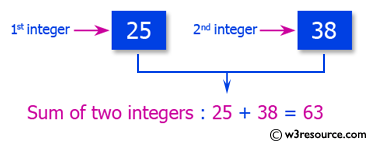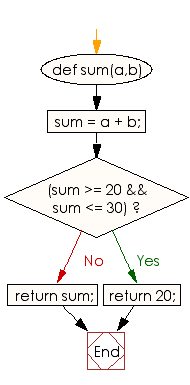﻿ Ruby Basic exercises: Compute and print the sum of two given integers - w3resource# Ruby Basic Exercises: Compute and print the sum of two given integers

## Ruby Basic: Exercise-44 with Solution

Write a Ruby program to compute and print the sum of two given integers, print 30 if the sum is in the range 20..30 (inclusive).Ruby Code:

``````def sum(a,b)
sum = a + b;
if(sum >= 20 && sum <= 30)
return 20;
end
return sum;
end
print sum(9, 7),"\n"
print sum(12, 10),"\n"
print sum(22, 15)
``````

Output:

```16
20
37
```

Flowchart:Ruby Code Editor: